Gauge your potential with Aakash BYJU'S JEE Main'23 Mock Test Gauge your potential with Aakash BYJU'S JEE Main'23 Mock Test

# JEE Main 2021 March 17 – Shift 1 Chemistry Question Paper with Solutions

JEE Main 2021 March 17th shift 1 Chemistry question paper can be viewed and downloaded from this page. Candidates will also find solutions for all the questions present in the JEE Main March session question paper. This will further help aspirants to analyze their performance in the JEE Main exam. JEE Main 2021 question paper for Chemistry (March 17th Shift 1) can be downloaded in the form of PDF below.

### JEE Main 2021 March 17th Shift 1 Chemistry Question Paper

SECTION-A

Question 1. The INCORRECT statement(s) about heavy water is (are)

(A) Used as a moderator in a nuclear reactor

(B) Obtained as a by-product in the fertilizer industry

(C) Used for the study of the reaction mechanism

(D) Has a higher dielectric constant than water

Choose the correct answer from the option given below:

1. a. (B) only
2. b. (B) and (D) only
3. c. (C) only
4. d. (D) only

Solution:

D2O = 78.06 (Dielectric constant)

H2O = 78.39 (Dielectric constant)

Question 2. Given below are two statements:

Statement I: Potassium permanganate on heating at 573 K forms potassium manganate.

Statement II: Both potassium permanganate and potassium manganate are tetrahedral and paramagnetic in nature.

In the light of the above statements, choose the most appropriate Ans from the options given below:

1. a. Both statement I and statement II are true
2. b. Both statement I and statement II are false
3. c. Statement I is true but and statement II is false
4. d. Statement I is false but statement II is true

Solution: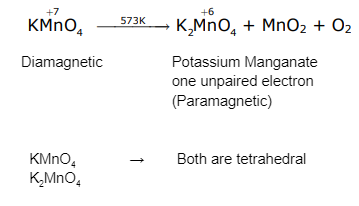Question 3. Which of the following is the correct structure of tyrosine?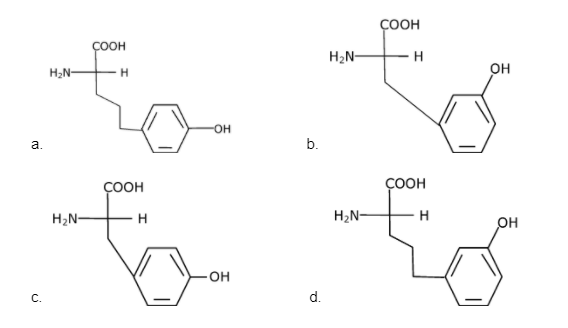Solution:

Fact.

Question 4. Given below are two statements:

Statement I: Retardation factor (Rf) can be measured in meter/centimetre

Statement II: Rf value of a compound remains constant in all solvents.

Choose the most appropriate answer from the options given below:

1. a. Statement I is false but statement II is true
2. b. Both statement I and statement II are false
3. c. Both statement I and statement II are true
4. d. Statement I is true but statement II is false

Solution:

Rf (Retardation factor is dimensionless)

Question 5. Mesityl oxide is a common name of:

1. a. 3-Methyl cyclohexane carbaldehyde
2. b. 4-Methyl pent-3-en-2-one
3. c. 2,4-Dimethyl pentan-3-one
4. d. 2-Methyl cyclohexanone

Solution: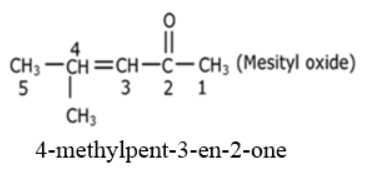Question 6. What is the spin-only magnetic moment value (BM) of a divalent metal ion with atomic number 25, in its aqueous solution?

1. a. 5.92
2. b. 5.26
3. c. Zero
4. d. 5.0

Solution:

25Mn — 1s2 2s2 2p6 3s2 3p6 4s2 3d5

spin – only magnetic moment = √n(+ 2) BM (n = 5)

= √5(5+2)

= √35

= 5.92 BM

Question 7. A central atom in a molecule has two lone pairs of electrons and forms three single bonds. The shape of this molecule is:

1. a. Trigonal pyramidal
2. b. T-shaped
3. c. See-saw
4. d. Planar triangular

Solution:

Steric number =2 L.P + 3 B.P ⇒ 5 (sp3d)

T-shapeQuestion 8. Product “A” in the below chemical reaction is: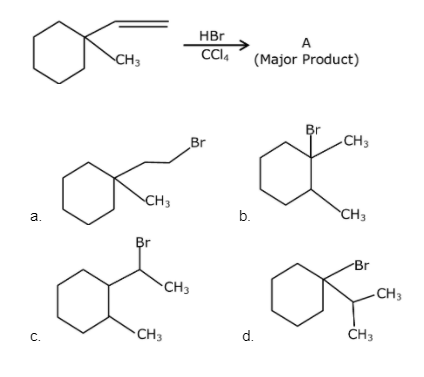Solution: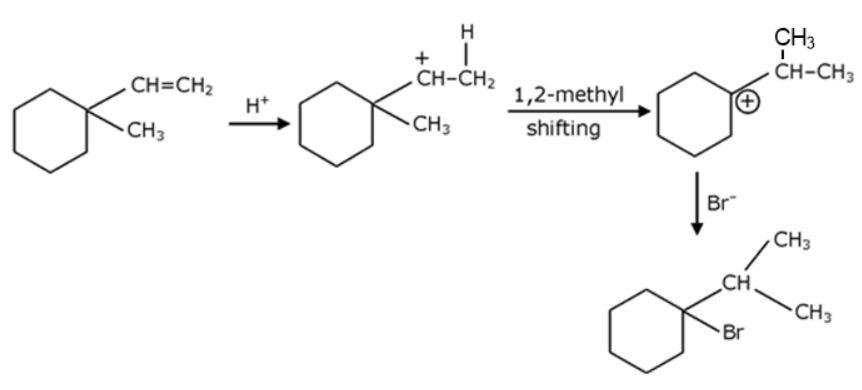Question 9. The point of intersection and sudden increase in the slope, in the diagram given below respectively, indicates: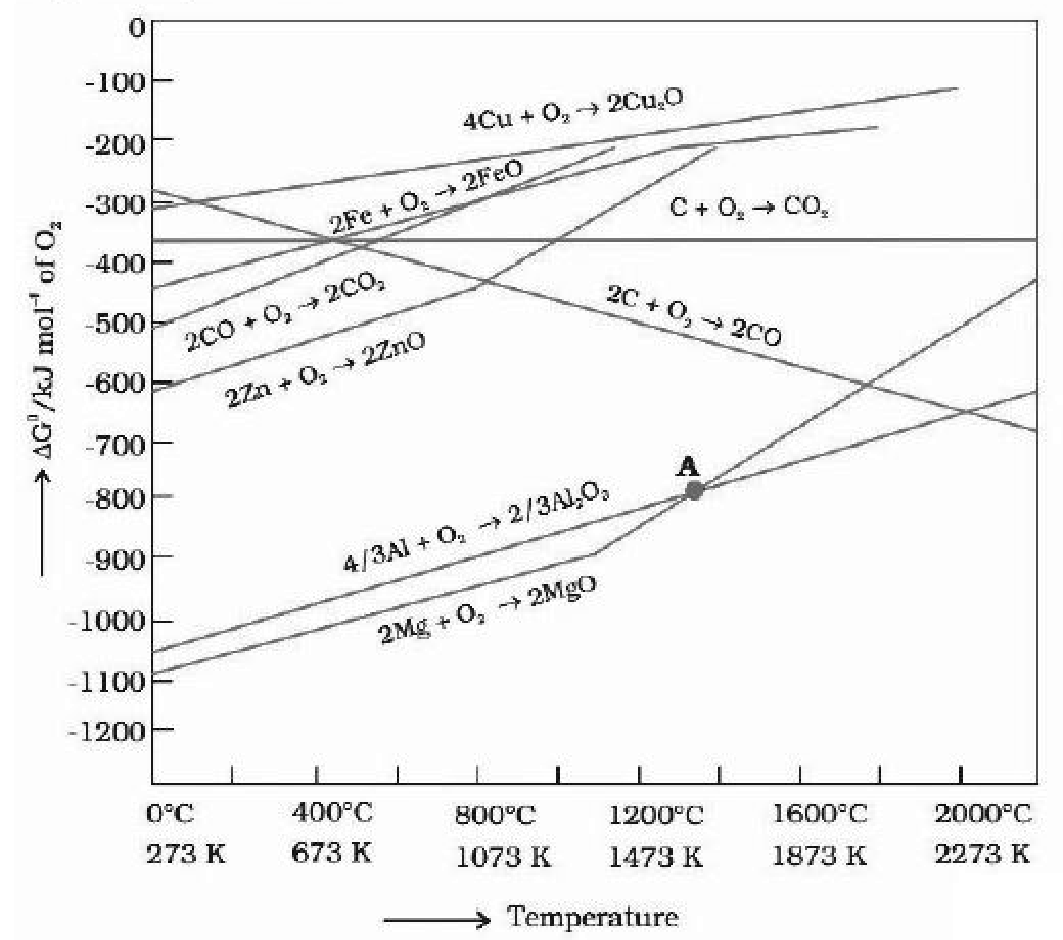1. a. ΔG = 0 and melting or boiling point of the metal oxide.
2. b. ΔG < 0 and decomposition of the metal oxide.
3. c. ΔG = 0 and reduction of the metal oxide.
4. d. ΔG > 0 and decomposition of the metal oxide.

Solution:

At the point of intersection ΔG = 0 for involved reaction.

Question 10. The reaction given below requires which of the following reaction conditions:1. a. 623 K, 300 atm
2. b. 573 K, 300 atm
3. c. 573 K, Cu, 300 atm
4. d. 623 K, Cu 300 atm

Solution:

The required conditions were 623 K and 300 atm.

Question 11. The correct order of conductivity of ions in water is:

1. a. Cs+>Rb+> K+> Na+
2. b. K+> Na+> Cs+>Rb+
3. c. Rb+> Na+> K+>Li+
4. d. Na+> K+>Rb+> Cs+

Solution:

Cs+(aq.) has a lower hydrated radius so its electrical conductivity is higher.

Question 12. A colloidal system consisting of a gas dispersed in a solid is called a/an:

1. a. Aerosol
2. b. Solid Sol
3. c. Foam
4. d. Gel

Solution:

 Dispersed phase Dispersion medium Type of colloid Gas Solid Solid Sol

Question 13. The absolute value of the electron gain enthalpy of halogen satisfies:

1. a. I > Br >Cl> F
2. b. F >Cl> Br > I
3. c. Cl> F > Br > I
4. d. Cl> Br > F > I

Solution:

Chlorine has higher electron gain enthalpy than fluorine due to less electron density.

Question 14. Which of the following reaction is an example of ammonolysis?

1. a. C6H5CH2CN → C6H5CH2CH2NH2
2. b. C6H5COCl + C6H5NH2 → C6H5CONHC6H5
3. c. C6H5CH2Cl +NH3 → C6H5CH2NH2
4. d. C6H5NH2 → C6H5NH3+Cl-

Solution:

C6H5CH2Cl +NH3 C6H5CH2NH2

Question 15. Reducing smog is a mixture of:

1. a. Smoke, fog and N2O3
2. b. Smoke, fog and O3
3. c. Smoke, fog and SO2
4. d. Smoke, fog and CH2=CH–CHO

Solution:

Reducing smog = smoke + fog + SO2

Question 16. Which of the following is an aromatic compound?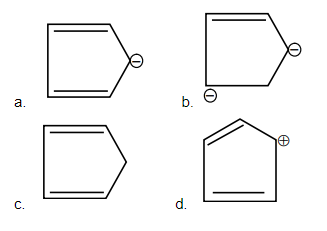Solution: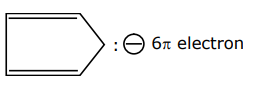Question 17. With respect to drug-enzyme interaction, identify the wrong statement.

1. a. Allosteric inhibitor competes with the enzyme’s active site
2. b. Competitive inhibitor binds to the enzyme’s active site
3. c. Non-competitive inhibitor binds to the allosteric site
4. d. Allosteric inhibitor changes the enzyme’s active site

Solution:

Lewis bases are electron donor and since PCl5 does not contain a lone pair therefore it cannot act as a lewis base.

Question 18. Hoffmann bromamide degradation of benzamide gives product A, which upon heating with CHCl3 and NaOH gives product B. The structures of A and B are: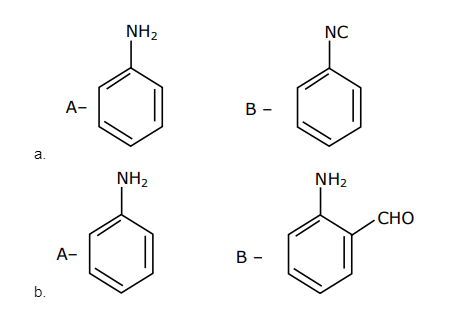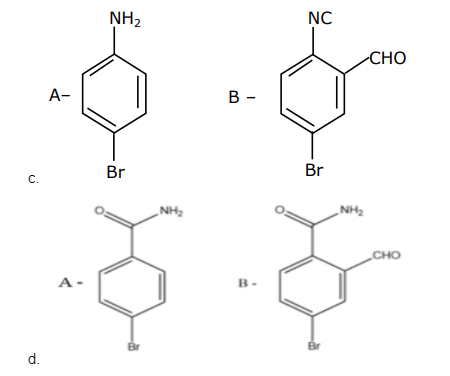Solution: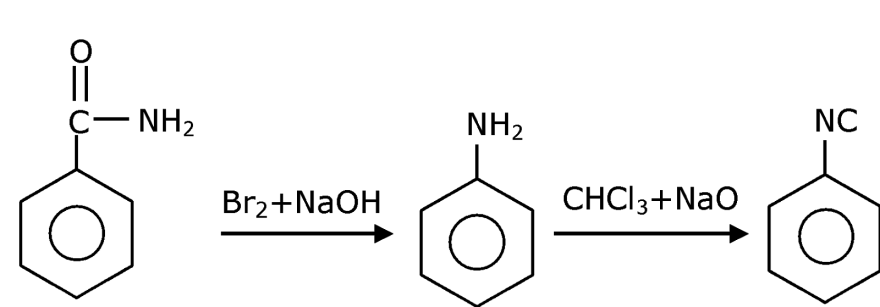Question 19. The product “A” in the above reaction is: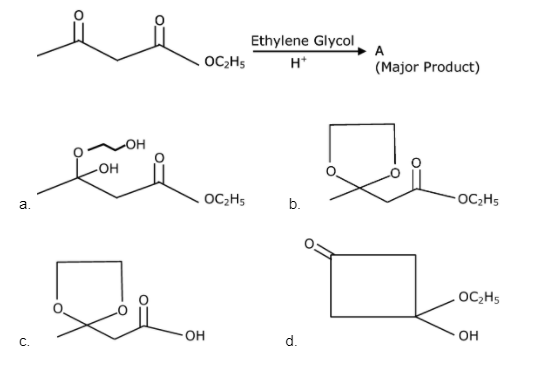Solution:Question 20. Which of the following compound CANNOT act as a Lewis base?

1. a. ClF3
2. b. PCl5
3. c. NF3
4. d. SF4

Solution:

NF3 has no vacant orbital neither in nitrogen nor in fluorine so it cannot accept the electron and hence, cannot acts as lewis acid and but for PCl5 P has no L.P & hence it cannot acts as a base but ClF3 (3 B.P + 2 L.P) & SF4 (4 B.P + 1 L.P)

Section-B

Question 21. A certain orbital has n = 4 and ml = –3. The number of radial nodes in this orbital is ____.(Round off to the Nearest Integer).

Solution:

Number of radial nodes = n – l– 1

n = 4, ml =–3 so l =3

radial nodes = 4 – 3 – 1 = 0

Question 22. 15 mL of an aqueous solution of Fe2+ in the acidic medium completely reacted with 20 mL of 0.03 M aqueous Cr 2O72-. The molarity of the Fe2+ solution is _____× 10–2M.(Round off to the Nearest Integer).

Solution:

By law of equivalence Meq of Fe2+ = Meq of Cr 2O72-

M × 15 × 1 = 0.03 × 6 × 20

M = 0.24 M = 24 × 10–2 M

Question 23. The reaction of white phosphorus on boiling with alkali in an inert atmosphere resulted in the formation of product ‘A’. The reaction of 1 mol of ‘A’ with an excess of AgNO3 in an aqueous medium gives _______ mol(s) of Ag. (Round off to the Nearest Integer).

Solution: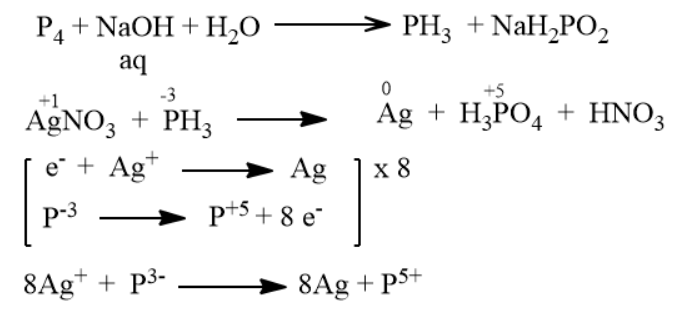So, the final reaction along with the stoichiometric coefficient is.

8AgNO3 + PH3 + 4H2O 8Ag + H3PO4 + 8HNO3

Excess 1 mol

Hence, 1 mol produce 8 mol Ag

Question 24. The oxygen dissolved in water exerts a partial pressure of 20 kPa in the vapour above water. The molar solubility of oxygen in water is _____ × 10–5 mol dm–3.
(Round off to the Nearest Integer).

[Given : Henry’s law constant = KH = 8.0 × 104 kPa for O2. Density of water with dissolved oxygen = 1.0 kg dm–3 ]

Solution:

P(g) = [KH]X

20 × 103 = [8.0 × 103 × 104] × Solubility

Solubility =

$$\frac{20\times 10^{3}}{8.0\times 10^{7}}$$

Solubility = 25 × 10–5 mol/dm3

Question 25. The standard enthalpies of formation of Al2O3 and CaO are –1675 kJ mol–1 and –635 kJ mol–1 respectively. For the reaction 3CaO + 2Al → 3Ca + Al2O3 the standard reaction enthalpy ΔrH0=______ kJ. (Round off to the Nearest Integer)

Solution:

ΔrH0f = ΔrH0f (Products) – ΔrH0f (Reactants)

= ΔrH0f(Al2O3)–3×ΔrH0f(CaO)

= – 1675 –3 (–635)

Question 26. For a certain first-order reaction 32% of the reactant is left after 570s. The rate constant of this reaction is _______ × 10–3 s –1. (Round off to the Nearest Integer).
[Given: log102 = 0.301, ln10 = 2.303]

Solution:

k = 1/t ln a / [a-x]

k = 2.303 / 570 log 100 / 32

k = 2.303 / 570 × 0.5

k = 2 × 10-3 s-1

Question 27. The pressure exerted by a non-reactive gaseous mixture of 6.4 g of methane and 8.8 g of carbon dioxide in a 10 L vessel at 27°C is _____ kPa. (Round off to the Nearest Integer). [Assume gases are ideal, R = 8.314 J mol–1 K –1 Atomic masses: C : 12.0u, H : 1.0u, O : 16.0 u]

Solution:

V = 10 L, T = 27° C = 300 K

(m)methane = 6.4 g, CO2 (m) = 8.8 g

PV = ntotalRT

P × 10–2 = (0.4 + 0.2) × 8.314 × 300

P = 149652 Pa ⇒ P = 149.652 kPa ≈ 150 kPa

Question 28. The mole fraction of a solute in a 100 molal aqueous solution is _____ × 10–2 . (Round off to the Nearest Integer). [Given : Atomic masses : H : 1.0 u, O : 16.0 u]

Solution:

Let weight of H2O = 1000 g

Moles of solute = 100

(mole)H2O = 1000/18

Mole fraction of solute =

$$\frac{mole\: of\: solute}{Total\: moles} = \frac{1800}{2800}$$

Xsolute = 64 × 10-2

Question 29. In the above reaction, 3.9 g of benzene on nitration gives 4.92 g of nitrobenzene. The percentage yield of nitrobenzene in the above reaction is _____%. (Round off to the Nearest Integer). (Given atomic mass : C : 12.0 u, H : 1.0 u, O : 16.0 u, N : 14.0 u)Solution:

Moles of C6H6 = 3.9/78 = 0.05 mol

Moles of C6H5NO2 = 4.92/123 = 0.04 mol

By conserving moles of carbon, mole of C6H5 NO2 Formed theoretically are 0.05 mol

%yield =

$$\frac{moles\: formed\: actually}{moles\: formed\: theoretically} = \frac{1800}{2800}\times 100$$

%yield =

$$\frac{0.04}{0.05}\times 100 = 80%$$

Question 30. 0.01 moles of a weak acid HA (Ka = 2.0 × 10–6 ) is dissolved in 1.0 L of 0.1 M HCl solution. The degree of dissociation of HA is_______ × 10–5 (Round off to the Nearest Integer). Assume degree of dissociation << 1

Solution: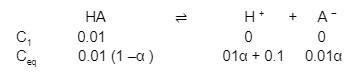$$K_{a}\frac{[H^{+}][A^{-}]}{[HA]}$$

$$2\times 10^{-6}=\frac{[0.1][0.01\alpha ]}{[0.01]}$$

α = 2 × 10–5

### JEE Main 2021 Chemistry Paper March 17 Shift 1